THE LAST TOTAL SOLAR ECLIPSE OF THE MILLENNIUM OVER ROMANIA
1999 August 11

PERSONAL OBSERVATIONS OF STEFAN BERINDE

• CLICK on each thumbnail to see an enlargement (640x480) of that picture!

• GO at the bottom of this page for more details! (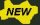updates posted on December 1999)

 THE REAL SCENARIO OF THIS ECLIPSE the sky is very clear, today it looks to be a normal hot summer day, do we have for sure an eclipse today? emotions increase... first contact 12h:43m:53s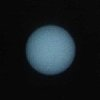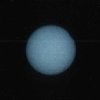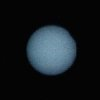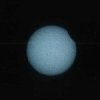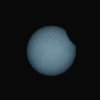01. C=0.1% T=12h:44m:25s EXP=1/500s+F 02. C=0.3% T=12h:45m:32s EXP=1/500s+F 03. C=0.5% T=12h:46m:10s EXP=1/500s+F 04. C=0.9% T=12h:47m:11s EXP=1/500s+F 05. C=2.4% T=12h:50m:08s EXP=1/500s+F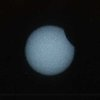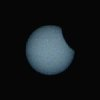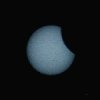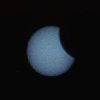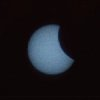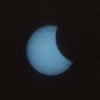06. C=3.0% T=12h:51m:06s EXP=1/500s+F 07. C=7.1% T=12h:56m:50s EXP=1/500s+F 08. C=11.5% T=13h:01m:48s EXP=1/500s+F 09. C=16.5% T=13h:06m:51s EXP=1/500s+F 10. C=21.9% T=13h:11m:52s EXP=1/500s+F 11. C=25.3% T=13h:14m:50s EXP=1/500s+F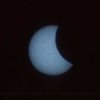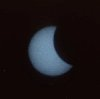sun becomes weaker, dogs start to bark, peoples are gathering to observe the sun, daylight decreases slowly.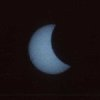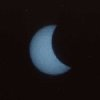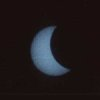12. C=30.8% T=13h:19m:22s EXP=1/500s+F 13. C=35.0% T=13h:22m:42s EXP=1/500s+F 14. C=41.4% T=13h:27m:33s EXP=1/500s+F 15. C=42.9% T=13h:28m:39s EXP=1/500s+F 16. C=49.0% T=13h:33m:10s EXP=1/500s+F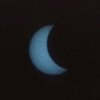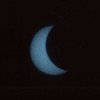wind starts to breeze, insects intensify their activity, sickles becomes visible under the trees, birds fly mainly around trees, the temperature at sun is still high.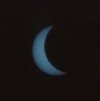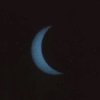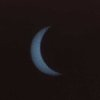17. C=53.8% T=13h:36m:33s EXP=1/500s+F 18. C=59.2% T=13h:40m:21s EXP=1/500s+F 19. C=67.8% T=13h:46m:16s EXP=1/500s+F 20. C=73.0% T=13h:49m:52s EXP=1/500s+F 21. C=74.1% T=13h:50m:34s EXP=1/500s+F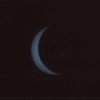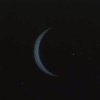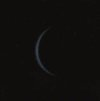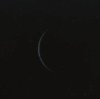small bevys of birds appear on the sky, the environement's luminosity decreases very quickly.22. C=81.2% T=13h:55m:16s EXP=1/500s+F 23. C=86.3% T=13h:58m:40s EXP=1/500s+F 24. C=91.5% T=14h:02m:08s EXP=1/500s+F 25. C=96.7% T=14h:05m:39s EXP=1/250s+F 26. C=98.6% T=14h:07m:01s EXP=1/250s+F venus appears shyly, the dark swallows us, peoples cheer, solar corona is outlining, light disappears in a diamond ring, second contact 14h:08m:19s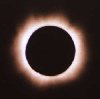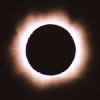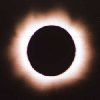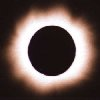outside is night, it seems to be cold, crickets sing, the horizon is yellow all over around, Baily's beads anchor the solar disk all the time, red protuberances are like hurts. 27. C=100% T=14h:08m:21s EXP=1/250s 28. C=100% T=14h:08m:25s EXP=1/125s 29. C=100% T=14h:08m:29s EXP=1/60s 30. C=100% T=14h:08m:34s EXP=1/30s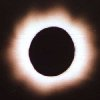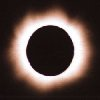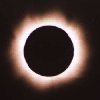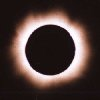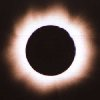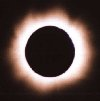31. C=100% T=14h:08m:40s EXP=1/30s 32. C=100% T=14h:08m:59s EXP=1/60s 33. C=100% T=14h:09m:02s EXP=1/125s 34. C=100% T=14h:09m:08s EXP=1/125s 35. C=100% T=14h:09m:58s EXP=1/30s 36. C=100% T=14h:10m:20s EXP=1/60s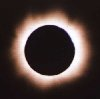from west a wall of light comes at high speed, the diamond ring appears instantaneoulsy, third contact 14h:10m:35s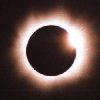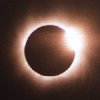the sunlight becomes blindly, we put back the sunglasses and filters, outside is daylight again, a little bit disappointment because it was to short.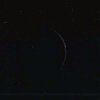37. C=100% T=14h:10m:27s EXP=1/125s 38. C=100% T=14h:10m:34s EXP=1/125s 39. C=99.9% T=14h:10m:38s EXP=1/250s 40. C=97.9% T=14h:12m:19s EXP=1/250s+F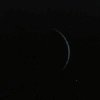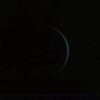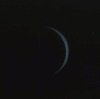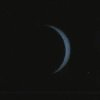birds fly again grouped in small bevys, outside is not hot yet and sun shines like in the morning, everybody has disappeared already from streets.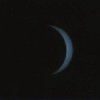41. C=96.7% T=14h:13m:07s EXP=1/250s+F 42. C=94.4% T=14h:14m:39s EXP=1/500s+F 43. C=90.9% T=14h:16m:52s EXP=1/500s+F 44. C=86.6% T=14h:19m:32s EXP=1/500s+F 45. C=82.0% T=14h:22m:27s EXP=1/500s+F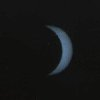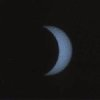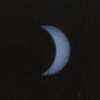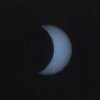the temperature at sun increases suddenly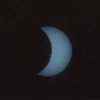46. C=72.9% T=14h:28m:14s EXP=1/500s+F 47. C=66.0% T=14h:32m:38s EXP=1/500s+F 48. C=63.3% T=14h:34m:25s EXP=1/500s+F 49. C=54.7% T=14h:40m:07s EXP=1/500s+F 50. C=49.3% T=14h:43m:49s EXP=1/500s+F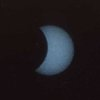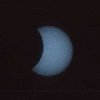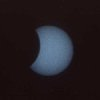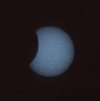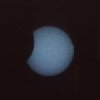the day becomes normal, it's very hot now, just the notched sun remebers us about the eclipse. 51. C=35.2% T=14h:53m:48s EXP=1/500s+F 52. C=28.6% T=14h:58m:49s EXP=1/500s+F 53. C=20.9% T=15h:05m:06s EXP=1/500s+F 54. C=15.5% T=15h:09m:51s EXP=1/500s+F 55. C=9.5% T=15h:15m:47s EXP=1/500s+F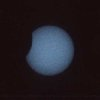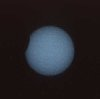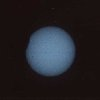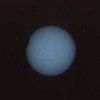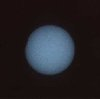for the naked eye the eclipse is over. 56. C=5.7% T=15h:20m:15s EXP=1/500s+F 57. C=1.9% T=15h:25m:48s EXP=1/500s+F 58. C=0.9% T=15h:27m:39s EXP=1/500s+F 59. C=0.7% T=15h:28m:16s EXP=1/500s+F 60. C=0.5% T=15h:28m:50s EXP=1/500s+F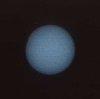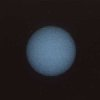last contact 15h:30m:48s ECLIPSE OVER! LEGEND C - solar disk coverage T - time (GMT + 3) EXP- exposure (F - with filter) 61. C=0.2% T=15h:29m:37s EXP=1/500s+F 62. C=0.2% T=15h:29m:44s EXP=1/500s+F 63. C=0.02% T=15h:30m:36s EXP=1/500s+F

I have posted below some details regarding